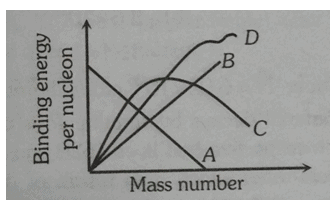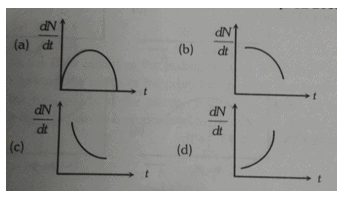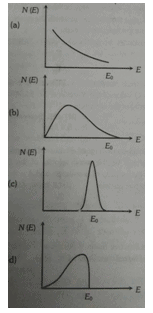Courses

# Atom And Nuclei - Online Test

## 30 Questions MCQ Test | Atom And Nuclei - Online Test

Description
This mock test of Atom And Nuclei - Online Test for Class 12 helps you for every Class 12 entrance exam. This contains 30 Multiple Choice Questions for Class 12 Atom And Nuclei - Online Test (mcq) to study with solutions a complete question bank. The solved questions answers in this Atom And Nuclei - Online Test quiz give you a good mix of easy questions and tough questions. Class 12 students definitely take this Atom And Nuclei - Online Test exercise for a better result in the exam. You can find other Atom And Nuclei - Online Test extra questions, long questions & short questions for Class 12 on EduRev as well by searching above.
QUESTION: 1

Solution:
QUESTION: 2

Solution:
QUESTION: 3

### Which of the following transitions in a hydrogen atom emits the photons of highest frequency ?

Solution:
QUESTION: 4

Half-life period of a radioactive element X is same as the mean-life time of another radioactive element Y. If initially both have same number of atoms, then

Solution:
QUESTION: 5

Activity of a radioactive material decreases to one third in 10 years. After 10 more years, final activity will be

Solution:
QUESTION: 6

Binding energy of deuterium is 2.23 MeV. Mass defect in amu is

Solution:
QUESTION: 7

When an electron jumps from the orbit n = 2 to n = 4, then wavelength of the radiations absorbed will be where R is Rydberg s constant

Solution:
QUESTION: 8

Half life of a radioactive substance is 10 second. Then undecayed nuclei of the substance will reduce to 6.25% of their value at t = 0 in a time of

Solution:
QUESTION: 9

Amount of original radioactive atom left after 5 half lives will be

Solution:
QUESTION: 10

the ratio of magnetic dipole moment to the angular momentum of the electron in the first orbit of hydrogen atom is

Solution:
QUESTION: 11

Hydrogen atom is excited from ground state to another state with principal quantum number equal to 4. Then the number of spectral lines in the emission will be

Solution:
QUESTION: 12

The magnetic moment (µ) of a revolving electron around the nucleus varies with principal quantum number n as

Solution:
QUESTION: 13

Which one of the following is not a possible energy for a photon to be emitted by hydrogen atom according to bohr’s atomic model

Solution:
QUESTION: 14

The radius of hydrogen atom in its ground state is 5.3 x 10-11 m. after collision with an electron it is found to have a radius of 21.2 x 10-11m. what is the principle quantum number n of the final state of the atom

Solution:
QUESTION: 15

First and second wavelength of Balmer series in hydrogen spectrum is (RH = 1.097 X 107 m)

Solution:
QUESTION: 16

The kinetic energy of electron in the first Bohr orbit of the hydrogen atom is

Solution:
QUESTION: 17

The ratio of minimum wavelengths of Lyman and Balmer series will be

Solution:
QUESTION: 18

When an α- particle of mass m moving with velocity bombards on a heavy nucleus of charge Ze, its distance of closest approach from the nucleus depends on m as

Solution:
QUESTION: 19

The wavenumber of last line of balmer series in hydrogen spectrum will be

Solution:
QUESTION: 20

Two nuclei have their mass number in the ratio of 1:3. The ratio of their nuclear densities would be

Solution:
QUESTION: 21

The correct order of ionizing capacity of α, β and γ rays is

Solution:
QUESTION: 22

A radioactive nucleus(mass number A and atomic number Z) emits 3 α particles and 2 positrons. The ratio of number of neutrons to that of proton in the final nucleus will be

Solution:
QUESTION: 23

A nucleus nXm emits one α and one β particle. The resulting nucleus is

Solution:
QUESTION: 24

Binding energy per nucleon plotted against the mass number for stable nuclei is shown in the figure. Which curve is correctSolution:
QUESTION: 25

. Radioactive element decay to form a stable nuclei then the rate of decay of reactant will vary with time as shown  in the figureSolution:
QUESTION: 26

The energy spectrum of β particles as a function of β energy emiited from a radioactive source isSolution:
QUESTION: 27

Which of the relation is correct between time period and number of orbits while an electron is revolving in an orbit

Solution:
QUESTION: 28

If scattering particles are 56 for 900 angle,then at an angle 600 will be

Solution:
QUESTION: 29

In the spectrum of hydrogen, the ratio of the longest wavelength in the Lyman series to the longest wavelength in balmer series

Solution:
QUESTION: 30

The wave number of a photon in bracket series of hydrogen atom is 9R/400. The electron has transited from the orbit having quantum number

Solution: